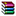2019-01-30 10:28:07

1下载字典和诗词

2训练

 A B C 1 =file("dict.btx").import@bi().keys(WORD).index() 2 =create(WORD,LEN,T) 3 宋词三百首宴山亭赵佶北行见杏花裁剪冰绡，轻叠数…… 4 =len(A3) >i=1 5 for (i<=A4) >word3=A2.find(mid(A3,i,3) ), word2=A2.find(   mid(A3,i,2) ), word1=A2.find( mid(A3,i,1) ) 6 if (word3!=null) >A2.insert(0, word3.(WORD),3,word3.(T)), i=i+3 7 else if (word2!=null) >A2.insert(0, word2.(WORD),2,word2.(T)), i=i+2 8 else if (word1!=null) >A2.insert(0, word1.(WORD),1,word1.(T)), i=i+1 9 else >i=i+1 10 =file("宋词模型.txt").export@t(A2)

A1读取字典文件，并且建立索引；

A2定义模板记录的数据结构，三个字段表示：词、长度、词性；

A5A9是循环处理，对A3进行分词，这里最长只处理三个字组成的词；

A10把结果保存；

3创作

 A B C 1 =file("宋词模型.txt").import@t() 2 =file("dict.btx").import@bi().keys(WORD).index() 3 明月几时有，把酒问青天。不知天上宫阙，今夕是何年。我欲乘风归去。惟恐琼楼玉宇，高处不胜寒，起舞弄清影，何似在人间。 4 =len(A3) >str="", i=1, words=null 5 for (i<=A4) >word3=A2.find(mid(A3,i,3)   ),word2=A2.find( mid(A3,i,2) ), word1=A2.find( mid(A3,i,1) ) 6 if (word3!=null) >words=A1.select(LEN==3&&   T==word3.(T) ), i=i+3, str=str+words(1+rand(words.len())).(WORD) 7 else if (word2!=null) >words=A1.select(LEN==2&&   T==word2.(T) ), i=i+2, str=str+words(1+rand(words.len())).(WORD) 8 else if (word1!=null) >words=A1.select(LEN==1&&   T==word1.(T) ), i=i+1, str=str+words(1+rand(words.len())).(WORD) 9 else >str=str+mid(A3,i,1), i=i+1 10 >output(str)15368090470002ffa.rar